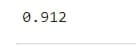## What is Geometric Distribution?

Geometric Distribution comes under discrete probability distribution that represents the probability of the number of successive failures before a success is obtained in a Bernoulli trial. There is only one possible outcome to a Bernoulli trial, i.e., success or failure. The probability of success is assumed to be the same for each trial.. Bernoulli trials are repeated until success is achieved in a geometric distribution and then stopped.

A geometric distribution is a discrete distribution for n = 0, 1, 2, ….. having probability density function. Geometric distributions are the only discrete memoryless random distributions. The geometric distribution resembles the exponential distribution. Using the geometric distribution, you can model the number of trials until a certain success or the number of failures until the first success. Either way, the sequence of probabilities is geometric.

In probability theory and statistics, geometric distribution is one of two discrete probability distributions. Which of these is called “the” geometric distribution is a matter of convention.

For clarity, however, it is recommended to explicitly refer to the support, thereby avoiding any ambiguity.A geometric distribution can be defined as a type of probability distribution that depends on three key assumptions and are as followed

• It is an independent trial.
• The outcome of each trial can only be either success or failure.
• Each trial has the same success probability, denoted by p.

As a discrete probability distribution, geometric distributions represent the probability of first achievement after a series of failures. The number of trials in a geometric distribution is indefinite until it yields a success.The sequence of probabilities is a geometric sequence.

#### Geometric Distribution FormulaA geometric distribution can be described by both the probability mass function (pmf) and the cumulative distribution function (CDF). The probability of success of a trial is denoted by p and failure is given by q. Here, q = 1 – p. A discrete random variable, X, that has a geometric probability distribution is represented as

X∼G(p)  X∼G(p)

Given below are the formulas for the pmf and CDF of a geometric distribution.

### We can define probability mass function as the probability that a discrete random variable, X, is exactly equal to some value, x. Here is the formula for the geometric distribution pmf:

P(X = x) = (1 – p)x – 1p

where, 0 < p ≤ 1.

### Probability that a random variable, X, with a value less than or equal to x, will come to be, at a point, x, is known as the cumulative distribution function. It is also called the distribution function. The formula for geometric distribution CDF is given as follows:

P(X ≤ x) = 1 – (1 – p)x

#### Mean of Geometric Distribution

The geometric distribution mean is also the expected value of the geometric distribution. A random variable, X, can be defined as the weighted average of all its values. A geometric distribution has the following formula for the mean:

E[X] = 1 / p.

Example:  For example, suppose an ordinary die is thrown repeatedly until the first time a “1” appears. The probability distribution of the number of times it is thrown is supported on the infinite set { 1, 2, 3, ….. } and is a geometric distribution with p = 1/6.

The geometric distribution is denoted by Geo(p) where 0 < p ≤ 1.

## The variance is a measure of dispersion that checks how far apart the data in a distribution are from the mean. A geometric distribution’s variance is calculated as follows:

Var[X] = (1 – p) / p2

## The standard deviation is equal to the square root of the variance. The standard deviation also shows the deviation of the distribution from the mean. The formula for the standard deviation of a geometric distribution is as follows:#### Coding implementation

Below we will see a small demonstration of geometric representation for distribution.

As you can see we have initialized the p and q variable with a value according to the formula mentioned earlier in order to get the distribution of probability on failure and success

				
val p = 1.0 / 3 // varible is declared with fraction


				
val q = 1.0 - p // similarly for variable q to see the probability of failure


				
var sum = 0.0



In this block we have used the formula to get the distribution as per our need and below that we have import decimal format to set the manner for our final decimal value.

				
// a condition statement for passing the value for finding the geometric distribution
for (n in 1..6) {
var a = 0.0
a = Math.pow(q, (n - 1).toDouble()) * p
sum += a
}


				
import java.text.DecimalFormat // import statement for the same


				
println(newFormat.format(sum)) // printing the newest format of value



#### OutputAbove you can see the output of the geometric distribution is presented which shows us the distribution among boolean values eg: success, failure.

Thank You so much for your valuable time. Hope you have learned something new and useful